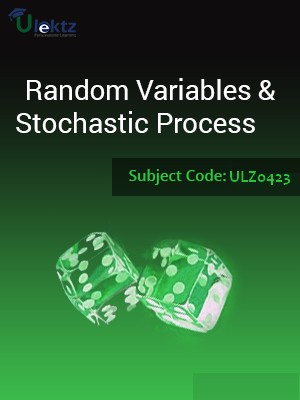•My WalletMy Order
•My Profile
•My Connections
•My Books
•My Videos
•My Tests
•My Calender
•My Messages
•My Shopping Cart
•My Orders
•Account Settings
•Help

# Book Details# Random Variables and Stochastic Process

 Course Code : ULZ0423 Author : uLektz University : General for All University Regulation : 2016 Categories : Electronics & Communication Format :ePUB3 (DRM Protected) Type : eBook

FREE

Description :Random Variables and Stochastic Process of ULZ0423 covers the latest syllabus prescribed by General for All University for regulation 2016. Author: uLektz, Published by uLektz Learning Solutions Private Limited.

Note : No printed book. Only ebook. Access eBook using uLektz apps for Android, iOS and Windows Desktop PC.

##### Topics
###### UNIT I THE RANDOM VARIABLES

1.1 The Random Variable: Introduction, Review of Probability Theory, Definition of a Random Variable - Conditions for a Function to be a Random Variable, Discrete, Continuous and Mixed Random Variables

1.2 Distribution and Density functions, Properties

1.3 Binomial, Poisson, Uniform, Gaussian, Exponential,Rayleigh, Conditional Distribution, Conditional Density, Properties

1.4 Rayleigh distribution

1.5 Conditional Distributions - Conditional density function

###### UNIT II OPERATION ON ONE RANDOM VARIABLE AND EXPECTATIONS

2.1 Operation on One Random Variable – Expectations: Introduction - Expected Value of a Random Variable - Function of a Random Variable

2.2 Moments about the Origin - Central Moments - Variance and Skew - Chebychev’s Inequality - Characteristic Function - Moment Generating Function

2.3 Transformations of a Random Variable - Monotonic Transformations for a Continuous Random Variable - Nonmonotonic Transformations of Continuous Random Variable

###### UNIT III MULTIPLE RANDOM VARIABLES AND ITS OPERATIONS

3.1 Multiple Random Variables: Vector Random Variables - Joint Distribution Function, Properties of Joint Distribution - Marginal Distribution Functions, Conditional Distribution and Density - Statistical Independence, Sum of Two Random Variables, Sum of Several Random Variables - Central Limit Theorem: Unequal Distribution, Equal Distributions

3.2 Operations On Multiple Random Variables: Joint Moments about the Origin, Joint Central Moments, Joint Characteristic Functions - Jointly Gaussian Random Variables: Two Random Variables case, N Random Variables case, Properties - Transformations of Multiple Random Variables - Linear Transformations of Gaussian Random Variables

###### UNIT IV RANDOM PROCESSES - TEMPORAL CHARACTERISTICS

4.1 Random Processes – Temporal Characteristics: The Random Process Concept - Classification of Random Processes - Deterministic and Nondeterministic Processes - Distribution and Density Functions

4.2 Concept of Stationarity and Statistical Independence - First-Order Stationary Processes, Second- Order and Wide-Sense Stationarity - Nth-order and Strict-Sense Stationarity - Time Averages and Ergodicity - Autocorrelation Function and its Properties - Cross-Correlation Function and its Properties - Covariance Functions - Gaussian Random Processes - Poisson Random Process

###### UNIT V RANDOM PROCESSES - SPECTRAL CHARACTERISTICS

5.1 Random Processes – Spectral Characteristics: The Power Spectrum: Properties - Relationship between Power Spectrum and Autocorrelation Function

5.2 The Cross-Power Density Spectrum, Properties - Properties of cross power density spectrum

###### UNIT VI LINEAR SYSTEMS WITH RANDOM INPUTS

6.1 Linear Systems With Random Inputs: Random Signal Response of Linear Systems - System Response – Convolution, Mean and Mean-squared Value of System Response - Autocorrelation Function of Response, Cross-Correlation Functions of Input and Output

6.2 Spectral Characteristics of System Response: Power Density Spectrum of Response - Cross-Power Density Spectra of Input and Output - Band pass, Band-Limited and Narrowband Processes, Properties

6.3 Modeling of Noise Sources: Resistive (Thermal) Noise Source, Effective Noise Temperature - Average Noise Figure, Average Noise Figure of cascaded networks